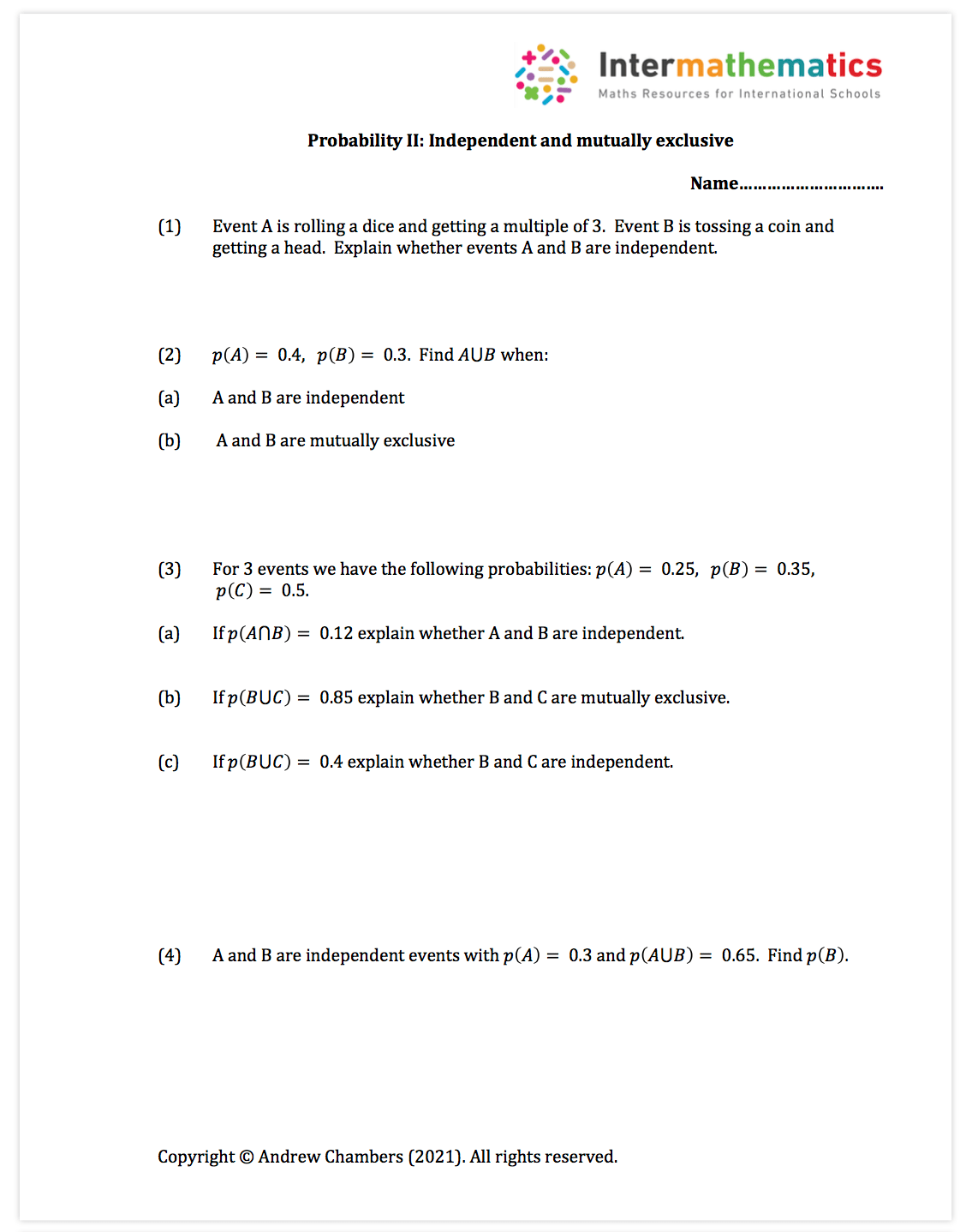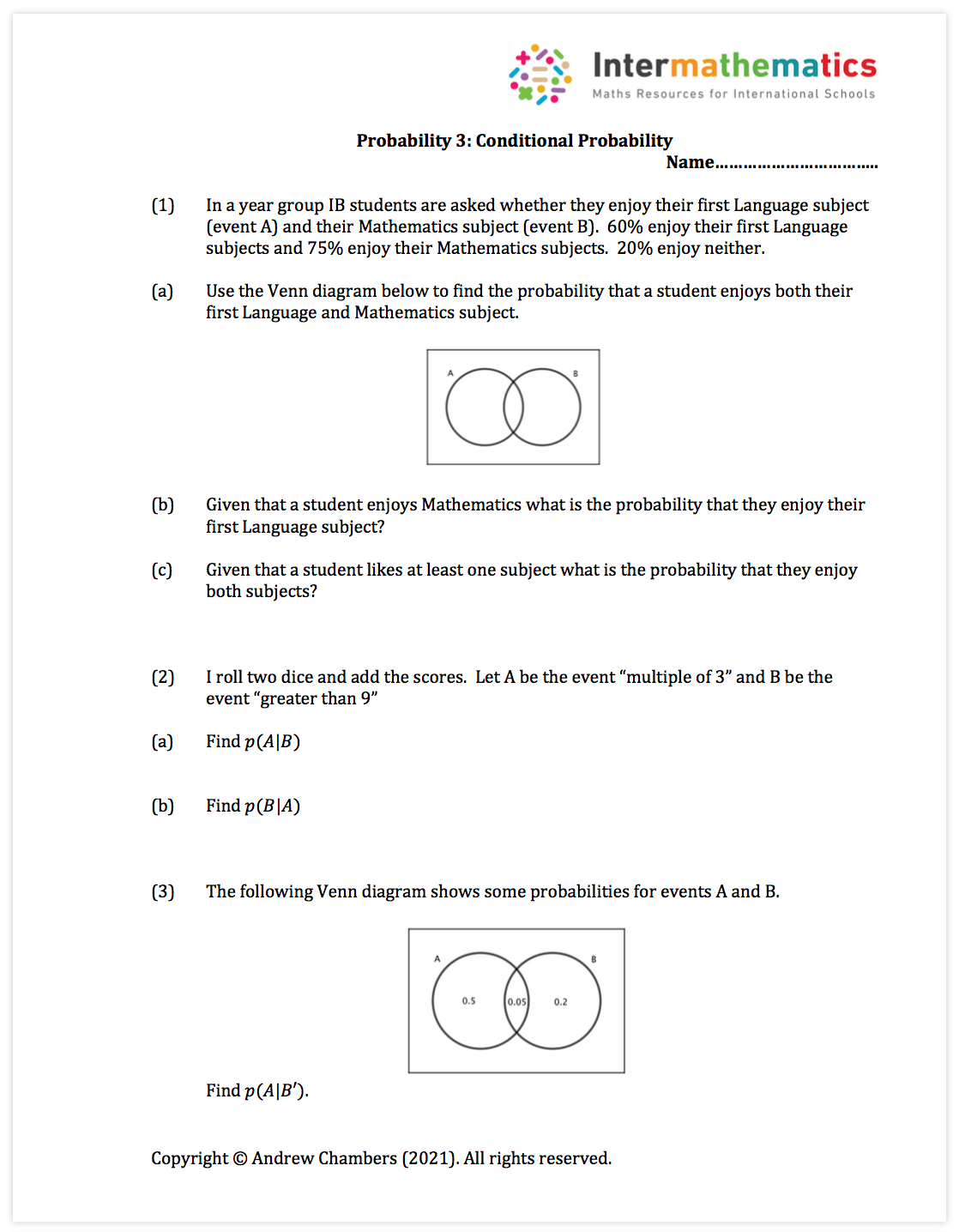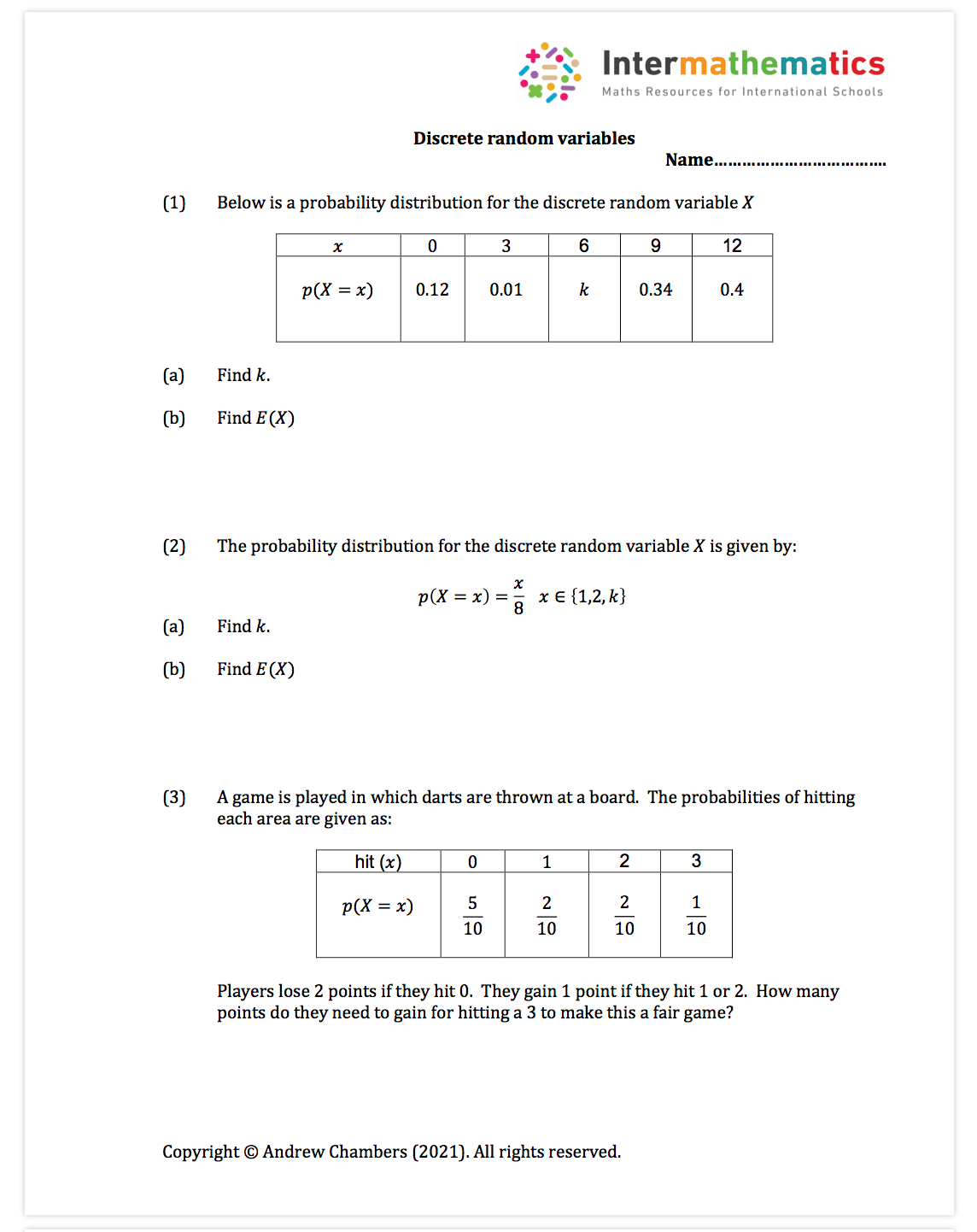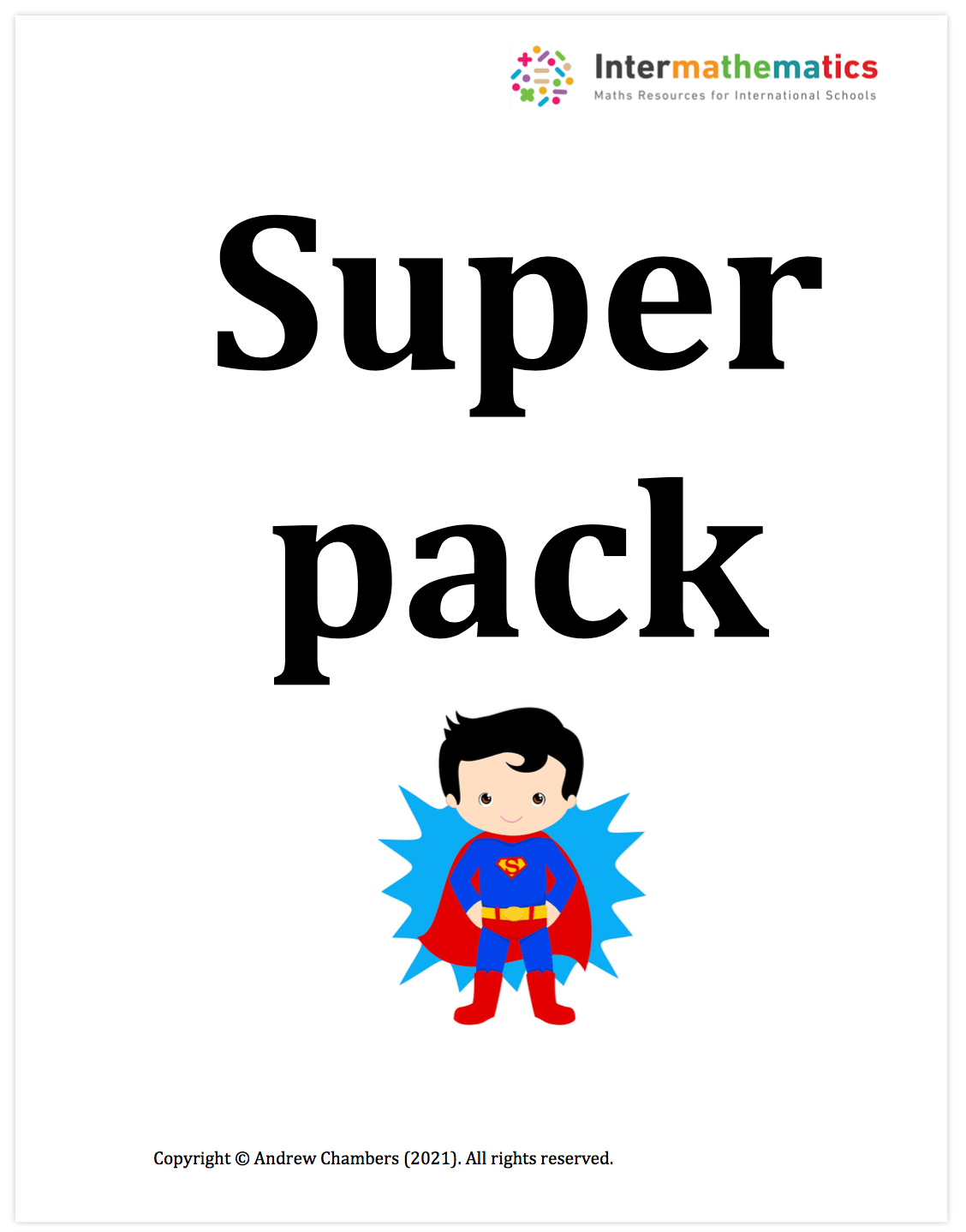# Applications: Probability

Statistics: Probability

Using and understanding probability – essential in modern life!

Syllabus content: SL 4.6, SL 4.7

I have made 5 worksheets and a Superpack to help with this:

1. Venn diagrams
2. Mutually exclusive and independent
3. Conditional probability
4. Discrete random variables
5. SuperPack: Conditional probability
6. Tree diagrams

You can scroll down below to see the pictures of the questions.  You can download both the questions and mark scheme from the bottom of the page.

1. Venn diagramsNote:  This is a short worksheet which can be used in lesson or for homework and gives some practice in basic Venn diagrams.  Syllabus point: SL 4.6.

2. Mutually Exclusive eventsNote:  This is a short worksheet which can be used in lesson or for homework and gives some practice in independence and mutual exclusiveness. Syllabus point: SL 4.6.

3. Conditional probabilityNote:  This is a short worksheet which can be used in lesson or for homework and gives some practice in independence and mutual exclusiveness. Syllabus point: SL 4.6.

4. Discrete random variablesNote:  This is a short worksheet which can be used in lesson or for homework and gives some practice in discrete random variables.  Syllabus point: SL 4.7.

5. Super Pack: Conditional ProbabilityNote: This is a bundle of fun resources for teaching conditional probability  – including treasure hunts, real life links to an investigation to Monty Hall and worksheets.  Lots of nice ideas for exploration topics!  Syllabus point: SL 4.6.

6. Tree diagramsNote:  This is a short worksheet which can be used in lesson or for homework and gives some practice in tree diagram questions.  Syllabus point: SL 4.6.